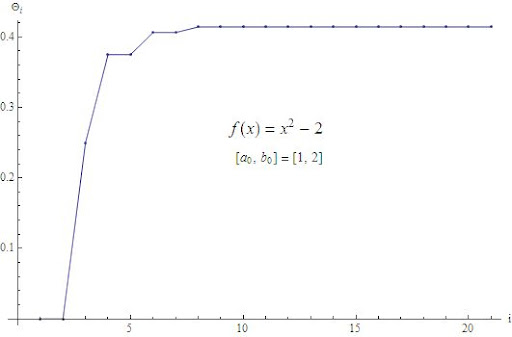## Saturday, 9 January 2010

### BINARY BISECTIONINTRO:

The Bisection Method is a very well-known root-finding algorithm that always comes at the very beginning of every book on Numerical Analysis.

The algorithm searches for a root in the interval, $latex [a_{0},b_{0}]$ in whose endpoints the continuous problem function, $latex f(x)$, takes opposite signs:

$latex f(a_{0})f(b_{0})<0$ The Bisection Method is based on Bolzano's Intermediate Value Theorem, and it can give, also, an alternate proof for it. (Reference )

Here, it does not matter function values: The algorithm does not use any other information from $latex f(x)$, other than its sign changes.

There are excelent pages on the internet that deal with this topic, (references ,  and  for example), and it would be useless to add more redundant and, for sure, worse presented summary about this numerical method.

I just want to take out something hidden inside the computer software, a small piece of math inside the programming area.

THE LOST FUNCTION

The core of the Bisection Method is the conditional IF... THEN... sentence, where the algorithm chooses in which half of the interval is the desired root.

This conditional evaluation can be converted into a decision function, Let's say $latex \delta_i$, gives $latex 0$ if the root is on the left half interval and $latex 1$, otherwise.

Now the iterative sequence can be rewritten using this function, $latex \delta_i$, where:

$latex m_{i}$ is the midpoint of the search interval:

$latex \displaystyle m_{i}=\frac{a_{i}+b_{i}}{2}$

$latex \delta_{i}$ is the decision function, and the $latex \delta(i,j)$, in the second member, is the Kronecker's delta:

$latex \delta_i=\delta(sgn(f(m_{i})),sgn(f(a_{i})))$

Then:

$latex \delta_{i} = \left\{ \begin{array}{ll} 1 & \mbox{if } (sgn(f(m_{i})=sgn(f(a_{i})) \\\\ 0 & \mbox{if } (sgn(f(m_{i})\neq sgn(f(a_{i})) \end{array}$

The endpoints of the search interval must be:

$latex {[a_{i+1}, b_{i+1}]} = \left\{ \begin{array}{ll} {[m_{i},b_{i}]} & \mbox{if }(\delta_{i}=0) \\\\ {[a_{i},m_{i}]} & \mbox{if } (\delta_{i}=1) \end{array}$

Then, they can be expressed with the aid of $latex \delta_{i}$, as:

$latex a_{i+1}=a_{i}+\delta_{i}\cdot (m_{i}-a_{i})$

$latex b_{i+1}=b_{i}+(1-\delta_{i})\cdot (m_{i}-b_{i})$

Note:
If $latex f(x)$, has only a single root in the interval $latex [a_{0},b_{0}]$, then it is possible to use an alternate version of the decision function:

$latex \delta_i=\delta(sgn(f(m_{i})),sgn(f(a_{0})))$

with the advantage that then it is not necessary to evaluate $latex f(a_{i})$ in the main loop of the algorithm: this implies an improvement in performance.

LENGTHS RATIO:

We can name lengths ratio between intervals $latex [a_{0},a_{i}]$, and $latex [a_{0},b_{0}]$, as the parameter:

$latex \theta_{i}=\frac{a_{i}-a_{0}}{b_{0}-a_{0}}$

If $latex \theta_{i}$ is expressed as a function of $latex \delta_{i}$:

$latex \displaystyle \theta_{i}=\sum_{k=0}^{i-1} \frac{\delta_{k}}{2^{k+1}}$

The sequence formed by the distinct values of the decision function, $latex \delta_{i}$, matches up with the binary expansion (or the binary digits) of $latex \theta_{i}$

Then the midpoint and the endpoints of the interval in function of $latex \theta_{i}$, are:

$latex a_{i}=a_{0}+(b_{0}-a_{0})\cdot \theta_{i}$

$latex \displaystyle m_{i}=a_{0}+(b_{0}-a_{0})\cdot \left(\theta_{i}+\frac{1}{2^{i+1}}\right)$

$latex \displaystyle b_{i}=a_{0}+(b_{0}-a_{0})\cdot \left(\theta_{i}+\frac{1}{2^{i}}\right)$

HACKING BISECTION METHOD:

The connection between $latex \delta_{i}$ and $latex \theta_{i}$ can be used to turn Bisection Method into a method to find the binary expansion of a root of any function that holds with theorem hypothesis.

For example if we take:

$latex f(x)=x^2-x_{0}$, where $latex x_{0}>0$

with $latex a_{0}=0$ and $latex b_{0}=2^{k}$, where $latex 2^{k-1} < \sqrt{x_{0}} < 2^{k}$, then: $latex \sqrt{x_{0}}=\lim_{i \to +\infty}{\theta_{i}}$ and the sequence of $latex \delta_{i}$ gives the binary digits of $latex \sqrt{x_{0}}$
Archives:

References:

-Mohamed A. Khamsi, Helmut Knaust - SOSMATH - The Bisection Method
-John H. Mathews - California State Univ. Fullerton - Module for The Bisection Method
-Holistic Numerical Methods Institute- - Bisection Method: Nonlinear Equations